# Theory of relativity

In physics, the theory of relativity is a scientific theory describing the effects due to the invariance of the speed of light. In particular, the meaning of space and time are altered by the motion of the observer. Relativity proposes time dilation and length contraction for observers moving relative to one another at very high ("relativistic") speeds.

Relativity refers to two closely-related mathematical theories in physics:*"I do not share the crusading spirit of the professional atheist whose fervor is mostly due to a painful act of liberation from the fetters of religious indoctrination received in youth. I prefer an attitude of humility corresponding to the weakness of our intellectual understanding of nature and of our own being." - Albert Einstein
• General Relativity (GR) is a theory to explain the laws of motion as viewed from accelerating reference frames and includes a geometric explanation for gravity. This theory was originally developed by Einstein, with help from David Hilbert in its final mathematical formulation, as a generalization of the postulates of Special Relativity to account for non-inertial, accelerating observers, particularly those in a gravitational field. A dramatic but later discredited claim by Sir Arthur Eddington of experimental proof of General Relativity in 1919 popularized the theory.

These theories have augmented earlier approaches, such as Galilean Relativity.

The theory of relativity is defended with religious-like zeal, such that no college faculty tenure, Ph.D degree, or Nobel Prize is ever awarded to anyone who dares criticize the theory, as the example of denying a Nobel Prize to the most accomplished physicist of the 20th century, Robert Dicke, illustrates. Other critics of the theory are Nikola Tesla, who called it a "...magnificent mathematical garb which fascinates, dazzles and makes people blind to the underlying errors. The theory is like a beggar clothed in purple whom ignorant people take for a king ... its exponents are brilliant men but they are metaphysicists, not scientists..." and Louis Essen [1908-1997], the man credited with determining the speed of light. He wrote fiery papers and many feature articles of the subject in science journals with popular audiences against it such as "Relativity and Time Signals" and "Relativity - Joke or Swindle?". Perhaps the most famous website opposing relativity is this one, with its Counterexamples to Relativity page. The cornerstone item in that page involves the experimental measurements of the advance of the perihelion of Mercury that show a shift greater than predicted by Relativity, well beyond the margin of error.

There are some issues of continuity and limits arising in the mathematical analysis of the theory of relativity. See Counterexamples to Relativity for discussion of this point.

The theory of relativity consist of complex mathematical equations relying on several hypotheses. For example, at Hofstra University general relativity is taught as part of an upperclass math course on differential geometry, based on three stated assumptions. Special relativity assumes that all observers in inertial frames of reference will measure the same value for the speed of light, c and that all inertial frames of reference are equivalent. These hypotheses that can never be fully tested. Relativity rejects Newton's action at a distance, which is basic to Newtonian gravity and also found to be a consequence of quantum mechanics. The mathematics of relativity assume no exceptions, yet in the time period immediately following the origin of the universe the relativity equations could not possibly have been valid, since quantum effects would not be negligible (in the same way non-relativistic quantum mechanics is not valid when dealing with particles traveling near the speed of light).

The "continuous" nature of space and time postulated by relativity is in conflict with the "discrete" nature in quantum mechanics, and although theories like string theory and quantum field theory have attempted to unify relativity and quantum mechanics, neither has been entirely successful or proven.

Unlike Newtonian physics, in which space and time intervals are each invariant as seen by all observers, in SR the only invariant quantity is a quadratic combination of space and time intervals (x2 - c2 t2). The instantaneous transmission of Newtonian gravitational effects also contradicts relativity.

In quantum mechanics, the uncertainty principle suggests that virtual particles can sometimes travel faster than the speed of light which would violate causality, but "[t]he only known way to resolve this tension involves introducing the idea of antiparticles." Consequently, in 1928 Paul Dirac derived the Dirac equation, one of the first quantum mechanical equations compatible with special relativity, by which Dirac predicted the existence of antimatter. Four years later, antimatter (the positron) was discovered by Carl Anderson, as successfully predicted by relativistic quantum mechanics. Quantum field theory, a generalization of quantum mechanics, is fully compatible with special relativity but not with general relativity, and still lacks a vital piece: evidence of the graviton.

## Special Relativity

Lorentz and Poincaré developed Special Relativity as way of understanding how Maxwell's equations for electromagnetism could be valid in different frames of reference. Einstein famously published an explanation of Poincaré's theory in terms of two assumptions (postulates):

1. The laws of physics are identical in all inertial reference frames.
2. The speed of light is the same for all (inertial) observers, regardless of their velocities relative to each other.

As an example of the first of these, if one performs an experiment—say, with a pendulum or some similar apparatus—in a railroad car traveling uniformly at 50 miles per hour, one would find the results of the experiment (positions, speeds, etc.) to be consistent with the laws of physics in the railroad car. An outside stationary observer, looking through the window, would also find the results consistent with the laws of physics in his stationary reference frame. He would simply have to subtract 50 miles per hour from the velocities of the objects in order to get values that agree with the values obtained by the observer on the train. But the two observations would each be consistent with the laws of physics. This is all straightforward and obvious to modern people, and has been since the time of Galileo. This principle is now called "Galilean relativity". Another way of stating this is:

• There is no "preferred" frame of reference.

Neither observer can claim that his frame of reference is "right". Of course, the observer on the train would think that the Earth is moving at 50 miles per hour under the wheels.

The second postulate is what makes relativity what it is. Using the above principle, if someone in the railroad car shines a beam of light forward, he would observe it moving at a speed of 670,000,000 miles per hour. One would expect, according to the first postulate, that an observer standing on the ground would measure its speed as 670,000,050 miles per hour. But the second postulate says that the stationary observer measures the same speed, 670,000,000 miles per hour. While this seems paradoxical, many experiments, such as the Michelson-Morley experiment show that it is nevertheless true. Special Relativity explains the apparent inconsistency between these postulates.

Why was this not observed until around 1900? Because the speed of light is so great that the discrepancy is very hard to measure. For example, in the railroad experiment described above, the discrepancy between the two speeds is 7.5 parts per million.

There are many far-reaching consequences of this, and many experimental verifications, some of which are listed below. Two of the more interesting consequences are that:

1. No material object of nonzero mass can travel at or greater than the speed of light. (Objects of zero mass, such as photons, travel at exactly the speed of light.)
2. Information can not travel faster than the speed of light. This is sometimes stated as:
3. There is no "action at a distance," because that would make observations dependent on the frame of reference. See Action at a distance for a discussion of this point.

When the assumptions are stated clearly as above, the weaknesses in the theory are more apparent. There “is” action at a distance in quantum entanglement and apparently also in gravity, as no gravitons can be found. However, no information has yet been transmitted via quantum entanglement, so while non-locality violates the spirit of relativity it is consistent with it if relativity is limited to the transmission of information. Quantum field theory, an attempt to partially reconcile quantum mechanics with relativity, is incomplete at best. As to the second assumption, it is contrary to the arrow of time, which illustrates the lack of symmetry in time. Logical defects include the incoherence of relativistic mass (see discussion below) and the lack of relativistic constraints near the beginning the universe (see above).

Special Relativity (SR) was initially developed by Henri Poincaré and Hendrik Lorentz, working on problems in electrodynamics and the Michelson-Morley experiment, which had not found any sign of Earth's orbital motion through the luminiferous aether, which was believed to be the substance which carried electromagnetic waves. Special relativity alters Isaac Newton's laws of motion by assuming that the speed of light will be the same for all observers, despite their relative velocities and the source of the light. (Therefore, if A sends a beam of light to B, and both measure the speed, it will be the same for both, no matter what the relative velocity of A and B. In Newtonian/Galilean mechanics, If A sends a physical object at a particular velocity towards B, and nothing slows it, the velocity of the object relative to B depends on the velocities of the object and of B relative to A.)

At low speeds (relative to light-speed), the Lorentz-Poincaré relativity equations are equivalent to Newton's equations. The media-promoted equation E=mc², implausibly suggests a relationship between typically unrelated concepts of energy, the rest mass of a body and the speed of light.

Under relativity, particles at low mass and low speed can be accurately approximated by classical mechanics (such as Isaac Newton's laws of motion). At the two extremes, modeling the behavior of electrons requires that relativistic effects be taken into account (the chemically significant phenomenon of electron spin arises from relativity), and the course of light passing through a region containing many massive bodies such as galaxies will be distorted (classical mechanics, in which light travels in straight lines, does not predict this). These are both experimentally confirmed (electron spin was known before relativity arose, and telescopic observations confirm that galactic clusters distort the paths of the light passing through them).

Many scientists have indicated problems with the postulates of special relativity. Paul Davies, formerly of Macquarie University and now at the University of Arizona believes that the speed of light has changed over time. Since the speed of light is a constant speed 'c' this indicates problems with the theory light speed. Other engineers and scientists have written about problems in the basic set of special relativity equations. Based on the ideas of not Einstein but of the scientist Fitzgerald as well as others, a length contraction effect was predicted as an explanation of the failure of the Michelson-Morley experiment to detect Earth's orbital motion. This idea was taken up by Hendrik Lorentz and shown by others to be a useful mechanism by which theory could be derived from experimental results. In fact, the Michelson-Morley experiment is often cited in textbooks as the derivation of relativity, though there are many other experiments that do this; see below.

In 2005, Michael Strauss, a computer engineer, claimed to have shown clear contradictions in Special Relativity, invalidating much of it. In 2004 he wrote the book Requiem for Relativity (The Collapse of Special Relativity), ASIN 0976312506. An archived ad for the book may be found at . The original web site for it is no longer active.

## General Relativity

See the General theory of relativity page for more in-depth coverage of this topic.

General Relativity is a theory of gravity that is compatible with Special Relativity. Einstein explains a thought experiment involving two elevators. The first elevator is stationary on the Earth, while the other is being pulled through space at a constant acceleration of g. Einstein realized that any physical experiment carried out in the elevators would give the same result. This realization is known as the equivalence principle and it states that accelerating frames of reference and gravitational fields are indistinguishable. General Relativity is the theory of gravity that incorporates Special Relativity and the equivalence principle.

General Relativity is a mathematical extension of Special Relativity. GR views space-time as a 4-dimensional manifold, which looks locally like Minkowski space, and which acquires curvature due to the presence of massive bodies. Thus, near massive bodies, the geometry of space-time differs to a large degree from that of other parts of the universe. Just as in classical physics, objects travel along geodesics in the absence of external forces. Importantly though, near a massive body, geodesics are no longer straight lines. It is this phenomenon of objects traveling along geodesics in a curved spacetime that accounts for gravity.

The "anomalous precession" of Mercury's perihelion (slow shift of the orbit's major axis) is explained by the Theory of General Relativity, but not by Newtonian mechanics. Some precession (5600 arcseconds per century) is caused by purely classical phenomena, mostly the gravitational attraction of other planets. But a small amount (43 arcseconds per century) of the observed precession, goes beyond that which classical Newtonian mechanics can explain. General relativity explains it accurately. There was another explanation based on Newtonian gravity, involving a slight alteration to the precise inverse-square relation of Newtonian gravity to distance, but it was quickly discarded when it gave very bad results for the Moon's orbit. The relativistic explanation of the anomalous precession is disputed on the Counterexamples to Relativity page of Conservapedia.

British Historian Paul Johnson declared the turning point in 20th century to have been when fellow Briton Sir Arthur Eddington, an esteemed English astronomer, ventured out on a boat off Africa in 1919 with a local Army unit to observe the bending of starlight around the sun during a total eclipse. Upon his return to England declared that his observations proven the theory of relativity. In fact recent analysis of Eddington's work revealed that he was biased in selecting his data, and that overall his data were inconclusive about the theory of relativity. The prediction was later confirmed by more rigorous experiments, such as those performed by the Hubble Space Telescope. Hendrik Lorentz had this to say on the discrepancies between the empirical eclipse data and Einstein's predictions.

It indeed seems that the discrepancies may be ascribed to faults in observations, which supposition is supported by the fact that the observations at Prince's Island, which, it is true, did not turn out quite as well as those mentioned above, gave the result, of 1.64, somewhat lower than Einstein's figure.

The bending of light by gravity is predicted both by Newtonian physics and relativity. Experimental observation agrees with the latter amount (1.75 arcseconds for the Sun), rather approximately for Eddington's measurements, and very accurately for more modern measurements.

Special relativity is the limiting case of general relativity where all gravitational fields are weak. Alternatively, special relativity is the limiting case of general relativity when all reference frames are inertial (non-accelerating and without gravity).

## Lack of evidence for Relativity

The Theory of relativity assumes that time is symmetric just as space is, but the biggest early promoter of relativity, Arthur Eddington, coined the term "arrow of time" admitting how time is not symmetric but is directional. The passage of time is tied to an increase in disorder, or entropy. The Theory of relativity cannot explain this, and implicitly denies it, specifically allowing for theoretical time travel (e.g., wormholes) and different rates of passage of time based on velocity and acceleration.

The Global Positioning System (GPS) requires exquisitely accurate timekeeping in the satellite clocks—down to the nanosecond. This precision is so great that the effects of both special relativity and general relativity play an important role. Without making corrections to the timing signals from the satellite clocks, the results of GPS measurements would be so far off that the system would be useless. The required amount of correction is well known, and the system makes those corrections. Those corrections are consistent with special relativity and general relativity, and it is generally accepted that this is no coincidence—relativistic effects are the reason why the corrections are necessary. But one doesn't need to believe that relativity is the reason the corrections are needed; one only needs to make the corrections in order to get the system to work.

Tom Van Flandern, an astronomer hired to work on GPS in the late 1990s, concluded that "[t]he GPS programmers don't need relativity." He was quoted as saying that the GPS programmers "have basically blown off Einstein." Asynchronization can be easily addressed through communications between the satellites and ground stations, so it is unclear why any theory would be needed for GPS. While Van Flandern believed that relativity is unnecessary for GPS, he also asserted that observations of GPS satellites supported both general and special relativity, writing that "we can assert with confidence that the predictions of relativity are confirmed to high accuracy over time periods of many days," with unrelated factors interfering with longer-term observations.

Some internet articles claim that GPS timing differences confirm the Theory of Relativity or its Lorentzian counterpart (which uses a preferred frame of reference). GPS clocks run slower in the weaker gravitation field of the satellites than on ground stations on Earth, with the effects predicted by general relativity far outweighing the effects predicted by special relativity. However, the articles claiming that the slower GPS satellite clocks confirm relativity do not address the effect, if any, of the weaker gravitational force under Newton's theory on the GPS satellite clocks, likely because in Newtonian Mechanics every clock in the universe keeps time at the same rate regardless of velocity, acceleration, or the presence or absence of force.

Currently, GPS satellites are synchronized to Coordinated Universal Time by radio signals from the ground; therefore, they cannot currently be used to test general relativity.

The effects of relativity have been observed with the frequency shift of the signal being sent back to Earth several times as various spacecraft have dipped into the gravity wells around massive objects such as the sun (see image at right) or Saturn. The Gravity Probe B satellite was put in orbit about the Earth to examine the effects of frame dragging and geodetic warping of space. One report was that it showed "unprecedented precision", and one showed a 95% confidence that the measured effect was consistent with General Relativity. Note, however, that one interpretation of Newtonian mechanics also predicts deflection of light by gravity. This is the "light is particles" interpretation, and gives a deflection of 0.875 arcseconds, whereas the "light is waves" interpretation predicts a deflection of zero. The experimentally measured deflection is 1.75 arcseconds, and is the value given by General Relativity (though Einstein's initial calculation was 0.875.).

None of the NASA spacecraft incorporates predictions of relativity into their own timing mechanisms, as Newtonian mechanics is adequate even for probes sent deep into space so long as they do not undergo accelerations near the speed of light or enter any massive gravity wells.

A decade of observation of the pulsar pair PSR B1913+16 detected a decline in its orbital period, which was attributed to a loss in energy by the system. It is impossible to measure the masses of the pulsars, their accelerations relative to the observers, or other fundamental parameters. Professors Joseph Taylor and Russell Hulse, who discovered the binary pulsar, found that physical values could be assigned to the pulsars to make the observed decline in orbital period consistent with the Theory of General Relativity, and for this they were awarded the 1993 Nobel Prize for Physics, which is the only award ever given by the Nobel committee for the Theory of Relativity. In 2004, Professor Taylor utilized a correction to the derivative of the orbital period to fit subsequent data better to the theory. At most, assumptions can be made and altered to fit the data to the theory, rather than the data confirming the theory.

The perihelion of Mercury's orbit precesses at a measurable rate, but even after accounting for gravitational perturbations caused all other planets in the solar system, Newton's theory (assuming a precise inverse-square relationship for distance) predicts a rate of precession that differs from the measured rate by approximately 43 arcseconds per century. While general relativity was developed on purely theoretical grounds, it was soon discovered that it explained these precession observations. Newton's theory can also explain the Mercury precession by making tiny adjustments to parameters in the gravitational equation, but doing so would give the same precession for all orbiting bodies everywhere, a phenomenon which is not observed.

General relativity predicts twice as much bending in light as it passes near massive objects than Newton's theory might predict. This phenomenon is known as gravitational lensing. A large number of instances of gravitational lensing have been observed, and it is now a standard astronomical tool. Note, however, that the extent of bending of light predicted by Newton's theory is open to debate, and depends on assumptions about the nature of light for gravitational purposes.

In 1972, scientists flew extremely accurate clocks ("atomic clocks") around the world in both directions on commercial airlines, and claimed to observe relativistic time dilation; the eastbound clock gained 273 ns and the westbound clock lost 59 ns, matching the predictions of general relativity to within experimental accuracy. However, the inventor of the atomic clock, Louis Essen, declared that the experiment was inaccurate. Dr A. G. Kelly examined the raw data from the experiment and declared it inconclusive. The Nobel Committee chose not to honor this experiment for the significance that was claimed.

## Experimental and Observational Evidence Confirming Relativity

The different effects predicted by special relativity, compared to classical formulations, are extremely tiny. Most relativistic effects are negligible at the speeds of ordinary phenomena observed by humans. The effects only become significant when the speeds involved are a significant fraction of the speed of light, which ismeters per second—such speeds are called relativistic. (However, it's worth noting that ordinary magnetism can be considered an effect of relativity, dictated by the need for electrostatic theory to be correct under relativity. The speed of light in fact appears in the formulas (Maxwell's Equations) governing electricity and magnetism, though these equations were developed long before relativity was proposed.)

Because the effects of relativity are so tiny, scientists have been devising sophisticated and sensitive tests ever since the theory was formulated in 1905.

It is important to be aware that it is fairly rare for an experiment to prove a theory. In general, experiments can only refute a theory. They can also be consistent with a theory. When enough experiments, especially experiments that investigate a wide variety of phenomena, are shown to be consistent with a theory, it lends credence to that theory. When no other plausible theory can explain those observations, we can say that they validate the theory. The Mercury observations are just one phenomenon. By themselves they couldn't validate or prove GR. And there was at one time another competing theory—the Newcomb-Hall exponent-fudging theory. That one didn't hold up for things other than Mercury. No other theory has come up explaining the phenomenon; SR and GR have withstood the test of time. That goes a long way toward validating them. There are many other observations and experiments, covering a wide variety of phenomena, described below—bending of light, gravitational time dilation, gravitational waves, geodetic precession, Shapiro effect, etc. It is these widely disparate observations, and the lack of any alternative theories explaining any of them, that lead people to say that SR and GR are "experimentally validated".

There's also the matter of simply "accepting" something, when a phenomenon is consistent with a theory that is already known to be valid. The recoil from a gun is an example of this. Everyone knows that there is a backward recoil when a gun is fired. This is explained to people when they are first being taught how to use a gun, and they get used to it with practice. The reason for the recoil is that it is an obvious consequence of Newtons' laws of motion. But people don't specifically think about Newton's laws, or consider this to be a "proof", when they are firing a gun. They just accept that the recoil is a known consequence of those laws. Newton's laws of motion have been validated through thousands of experiments much more focused than the firing of a gun.

The Cockcroft/Walton experiment is an example of this kind of acceptance. The results are consistent (to within 5% when analyzed carefully) with Special Relativity, and many people consider it to be a validation of SR. But there were too many other things going on, such as the use of the newly-invented particle accelerator, to make it a definitive test. One would have to think very carefully about what assumptions have to be made about what is happening in the experiment if one wanted to use it to assert anything about E=mc². However, famous as the experiment was at the time, it is no longer suggested as a validation of this formula. Special Relativity had already been validated through more focused and definitive tests. There are no known alternative theories, published in the scientific literature or listed in Counterexamples to Relativity, explaining the loss of mass. See Cockcroft and Walton Experiment.

The anomalous behavior of the timing signals from GPS satellites is another example. The phenomenon is well known—the system compensates for this; otherwise it wouldn't work—and the phenomenon is known to be a consequence of both special and general relativity. But the GPS system should not be considered and experiment to test relativity. No sensible person would build the GPS system as a test of relativity, if only because it exhibits two phenomena (time dilation of special relativity, 7 milliseconds per day, and time compression of general relativity, 45 milliseconds per day) that work against each other. No one would design an experiment that tests two phenomena working against each other.

The bending of light in a gravitational field is another example. (In fact, it was the first, and, for a while, most famous piece of evidence in favor of GR, even though it was sloppily done.) Newtonian physics also might predict the bending of light depending on whether one posits the "wave" formulation (deflection is zero) or the "particle" formulation (deflection is 0.875 arc seconds). But the actual deflection is 1.75 arc seconds, and GR predicts this value. The are no known versions of Newtonian gravity that get this larger value.

While the experimental tests for General Relativity are rather esoteric, those for Special Relativity are fairly straightforward. So much so that the Michelson-Morley experiment could be said to have single-handedly established the case for SR—the logical syllogism leading from Michelson-Morley to SR and the Lorentz transform was fairly clear once people saw it.

When analyzing an experiment to see whether it validates a theory, one must be careful not to assume the theory in one's reasoning. For establishing Special Relativity with the Michelson-Morley experiment, the assumptions are:

1. Newtonian and Galilean mechanics.
2. Galilean relativity, that is, the notion that there is no absolute frame of reference.
3. The universality of the speed of light.
4. And, of course, proper calibration of the equipment.
The third one was the observation that Michelson and Morley made.
For the derivation of E=mc², these assumptions are added:
5. Conservation of energy.
6. Conservation of momentum.
All of the assumptions listed above constitute SR.
It would be nearly inconceivable to derive GR without SR. Gravitational time dilation, for example, requires a lot of information coming from SR.
So, for the experiments listed below for GR, the assumptions are the 6 above plus:
7. The Equivalence Principle.
So the experiments below for GR are really just establishing that the Equivalence Principle is valid, and that GR follows logically from that.

The most famous experiment, and the one that is commonly cited in textbooks as the experiment that established the case for relativity, was the Michelson-Morley experiment. This showed that all observers will obtain the same measured value for the speed of light (3x108 meters per second) no matter what their state of motion. This is the first of the two fundamental principles:

1. The speed of light is constant for all observers, regardless of their velocities relative to each other.
2. The laws of physics are identical in all reference frames.

(The second is just a restatement of Galilean relativity, that is, the "common sense" that had been accepted for centuries.) A naive "common sense" interpretation of Galilean relativity would require that measurements of the speed of light (or anything else) by different observers would get results that differ by the observers' relative speeds, and hence that principle #1 can't be true. Special relativity fixes this apparent paradox.

All of special relativity derives for these two principles, plus assumptions of exact conservation of momentum and energy in all cases.

There are hundreds of experiments or observations that, to varying extents, "prove", "validate", or "are consistent with" special and general relativity. A good survey may be found at this site. Some experiments can be as simple as a spinning Alnico magnet and a voltmeter, that can be performed on a tabletop in a science classroom. Just a few of the more important ones will be described here.

### The Michelson-Morley experiment

This is the most famous experiment, and is often taken as the experiment establishing the correctness of special relativity. It is described elsewhere.

### Mass Change Associated With Energy Change

This is the famous formula "E=mc²". At the end of Einstein's original 1905 paper on the subject, he speculates on the possibility that the equation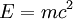, which would normally be very hard to verify, could be verified with the extremely high energies of the newly discovered phenomenon of radioactivity. In the 1910s, with the invention of the mass spectrometer, it became possible to measure masses of nuclei accurately. This led to the clearing up of the mystery of atomic masses not being exact integers,and strongly suggested the existence of a "mass defect" (or "packing fraction") consistent with the mass-energy equivalence. In the 1930s, experiments with known nuclear reactions showed a very accurate correlation between the masses of the nuclei involved and the energy released. See Quantitative Analysis of Alpha Decay.

### Time Dilation

Another prediction of special relativity was time dilation in rapidly moving objects. This effect was most famously verified in the anomalously slow decay of relativistic cosmic muons. Time dilation has since been verified many times, and is routinely taken into account in all high-energy nuclear physics experiments, as in Hadron collision experiments.

### The Cockcroft and Walton Experiment

This one is quite famous, because it was one of the earliest observations of phenomena involving mass change. But, as noted above, it can't really be taken as a "proof" of relativity.

### Bending of Light in a Gravitational Field

Unlike special relativity, predictions of general relativity turn out to be obscure and difficult to test. The two most famous predictions in the early days were the bending of light in a gravitational field and the precession of the perihelia of orbiting planets. Several other phenomena have arisen more recently.

The first of these was famously tested during a total eclipse in 1919. That test was somewhat muddled by an incorrect initial calculation, by several people including Einstein himself, of what the effect would be, and some "cherry picking" of the data to be used. The observed result was about 1.5 arc seconds. The data selection could be considered "manipulation" or "fudging", by a person (Arthur Eddington) who had a personal stake in the outcome. His analysis techniques would not pass muster today. The announcement of this test, flawed though it was, made Einstein world-famous.

It should be noted that pre-relativistic (Newtonian) physics may also predict a bending, depending on whether one uses the 17th century "corpuscular" formulation or the 19th century "wave" formulation. The former would give a value of 0.875 arc seconds, while the latter would give a value of zero.

Relying on the usual assumptions—Newtonian mechanics, Galilean relativity, conservation of energy and momentum, the universality of the speed of light (that is, special relativity), the Equivalence Principle, and the geodesic equation in empty space (that is, general relativity), and the proper calibration of the instruments, observations of later eclipses, and the observations of quasar 3C273, confirm predictions of the theory, which is that the bending is 1.75 arc seconds.

No Nobel Prize was awarded for this. A few years later Einstein won the Nobel Prize for the (unrelated) Photoelectric effect.

### Precession of the orbit of Mercury

The second "classical" test of general relativity was the advance of the perihelion of the orbit of Mercury. There are many complex effects contributing to this, including gravitational perturbations from other planets and the effect of the oblateness of the Sun. These are hard to calculate accurately, but, by 1860 it was known quite accurately that there was an "anomalous" precession, that is, a precession beyond all other known effects, of 43 arc seconds per century. This is a very tiny effect, but astronomical measurements were sufficiently accurate by that time to show it clearly. This was based on observations going all the way back to about 1700. It was calculated with great accuracy, and reported in 1859, by Urbain Leverrier. This was long before relativity was formulated.

One might wonder how astronomical observations accurate enough to measure such a tiny effect could have been made with the technology of that era. A clever trick was used: Solar transits of Mercury were observed and timed. Some of these transits were timed with an accuracy of about 15 seconds.
This created quite a problem—physicists by then were accustomed to having their theories check out very accurately. One proposal that was made, by Simon Newcomb and Asaph Hall, was that the exponent of the radius in the gravitational formula wasn't exactly 2. He showed that, by choosing an exponent of, the precession, as a fraction of a full orbit per planet's year, is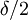. By setting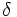to .000000157, that is, an exponent of 2.000000157, Newcomb was able to get a precession of .000000078 revolutions per Mercury year, or 43 arcseconds per Earth year. Whatever value is chosen for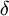, it gives the same precession, per revolution, for all orbiting bodies, but gravitational effects from other planets diminish that effect the further the planet is from the sun.
The approximation given at the end of Einstein's 1916 paper  is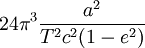revolutions per planet's "year", where a is the semi-major axis, T is the length of the planet's year, and e is the eccentricity. A much simpler, but less accurate, approximation, designed to show how the precession relates to the planet's speed, is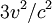revolutions per planet's "year", where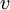is the planet's average orbital speed. These are just approximations. Getting an accurate value requires integrating the geodesic equation of the Schwartzschild metric, where Ricci's tensor is zero.
While Newcomb's theory, and general relativity, don't lead to closed-form solutions, both theories can be solved numerically to as much precision as one desires.
The following table shows some approximate parameters for the planets. Note that Mercury has the smallest orbit, and the fastest speed. Precession of planets other than Mercury is extremely hard to measure, but measurements of the actual anomalous precessions are in good agreement.
Planet Period, seconds x 106 Semimajor axis, meters x 109 Speed, meters/second x 103 Gravitational force, Newtons per kilogram Anomalous precession, arcseconds per (Earth) century, pure Newtonian mechanics Anomalous precession, Newtonian with exponent of 2.000000157 Anomalous precession, general relativity Measured anomalous precession (estimated uncertainty)
Mercury 7.57 58.9 48 .039 0 43 43 43.5(5)
Venus 19.6 108 35 .011 0 16.6 9 8(5)
Earth 31.6 150 30 .006 0 10.3 4 5(1)
Mars 59.3 227.9 24 .0025 0 5.5 1.4
Jupiter 374 778.4 13 .0002 0 0.87 0.07
Saturn 929 1426 9.7 .00006 0 0.35 0.014
Uranus 2651 2870 6.8 .000016 0 0.12 0.002
Neptune 5200 4498 5.5 .000007 0 0.063 0.0008
Considering only the anomalous precession, that is, the precession that remains after all known other factors (other planets and asteroids, solar oblateness) have been accounted for, and using very accurate calculations rather than the approximations given above, general relativity predicts 42.98 ±0.04 arcseconds per century. Some observed values, as of 2008, are:
43.11 ± 0.21 (Shapiro et al., 1976)
42.92 ± 0.20 (Anderson et al., 1987)
42.94 ± 0.20 (Anderson et al., 1991)
43.13 ± 0.14 (Anderson et al., 1992)
(Source: Pijpers 2008)
These error bars, and that of the general relativity prediction, all overlap.

Choosing a phenomenon to measure, and setting up an experiment to measure it, often benefits from a great deal of planning. For example, the Lee-Yang-Wu experiment that disproved parity in the weak force, took months to plan, and only about 15 minutes to conduct. Measurements of orbital precession due to general relativity are also aided by careful choice of the exact phenomena to measure.

The scientific community didn't have a lot of choice in how to measure the mechanical effects of general relativity in 1915. The only manifestation that was known at the time was the precession of planetary orbits. The orbit of Mercury was used because it has the highest precession of all the planets in the solar system. This is because it moves faster than any other planet. Though it is still nowhere near to being "relativistic", so the effect was extremely small.

1. The first question that is of interest is whether precession occurs, and how much. The precession of Mercury had been known, with considerable accuracy due to observations of transits of the Sun, for about 50 years. The precession does occur, and in very good agreement with theory. The data on this are discussed just above in this article.
2. We now have access to much better examples of orbits affected by general relativity, showing the effect much more strongly than could have been done in 1915. This is because orbiting bodies emit gravitational waves, which remove energy from the system and make the orbit decay. This effect is utterly impossible to observe in the case of Mercury's orbit, but it became possible with the discovery of pulsars in 1967. Binary pulsars are sufficiently massive, and orbit sufficiently closely, that their decay can be observed. This was observed for binary pulsar PSR B1913+16, and Joseph Taylor and Russell Hulse were awarded the 1993 Nobel Prize for this. The gravitational waves themselves were still far to weak to be observed directly.
3. By 2015, the LISA detector was able to observe gravitational waves directly, from decaying and colliding black holes and neutron stars.
4. In addition to the more recent discoveries involving much stronger "signals" than the observations of Mercury, those signals were much less contaminated by extraneous phenomena, or "noise". The calculated relativistic precession of Mercury is 43 arcseconds per (Earth) century, in the context of 5600 arcseconds per century of total precession. The latter effects are mostly due to the gravitational forces from other planets, 0.03 arcseconds from solar oblatenes, and 0.002 arcseconds from Lense-Thirring precession.

The orbits of stars near the black holes in galactic centers, while not showing decay or radiation, present a much stronger signal than Mercury. While the precession can't be quantified, it is still a very clear effect.

People have argued at great length (often here at Conservapedia) over the total precession discrepancy (about 1 arcsecond per century according to an article cited at Wikipedia) and relativity's role in it, but in fact there are other phenomena that are vastly better suited to testing Einsteinian gravity.

### The Shapiro EffectThe Shapiro effect: A spacecraft signal dipping into a gravity well around the Sun is delayed slightly.

Another is the Shapiro effect, involving time delay in radio signals passing through the gravity well of the Sun or a planet. Various spacecraft (such as Cassini) have confirmed this.

### Gravitational Time Dilation

Gravitational time dilation is an effect separate from the time dilation of special relativity. It was tested by the Pound-Rebka experiment in 1959. No Nobel Prize was awarded for this. Professor Pound had previously shared in the 1952 Nobel for his contributions to Nuclear Magnetic Resonance imaging.

### Gravitational Waves

Later in the 20th century, even more subtle phenomena were tested. One was the phenomenon of gravitational radiation, or "gravitational waves". These waves are incredibly difficult to observe, and had never been observed until 2015. But extremely dense binary pulsars radiate gravitational waves with sufficient energy loss that, even though we can't detect the waves from Earth, we can see the effect of the energy loss from the radiation. The extreme precision of the timing of pulses from pulsars makes it possible to observe their energy loss with great accuracy. Observations by Hulse and Taylor of the pulsar pair known as B1913+16, found the energy loss to be consistent with the predicted radiation. This required choosing orbital parameters to be consistent with observation, in the same way that Kepler chose orbital parameters to fit observed planetary motion to his theory. The rotating pulsars have since moved such that Earth is now out of the beams. The assumptions required for this, in addition to those listed above, were that pulsars behave consistently. The 1993 Nobel Prize in physics was awarded for this.

In late 2015 (and announced in 2016), the LIGO instruments directly detected gravitational waves allegedly from colliding black holes. See Gravitational waves. Unlike the waves from orbiting pulsars, that could only be detected by the loss of rotation energy, the waves from colliding black holes were strong enough to be detected directly. The assumptions required for this, in addition to those listed above, were that the objects emitting the radiation (generally believed to be black holes, of course) satisfy the Schwartzschild solution to relativistic mechanics. The 2017 Nobel Prize in physics was awarded for this.

Since that very early announcement, further observations of gravitational waves have continued to come in. In August 2019 the first detection of the gravitational waves from the merger of a black hole and a neutron star occurred. This was event S190814bv, 870 million light years away, and hence actually occurred 870 million years ago.

In 2018 an observation of the 3-body system PSR J0337+1715, consisting of 2 white dwarfs and a pulsar, was consistent with (and hence tended to confirm) general relativity under conditions of extremely high gravity, to enormous precision. The assumptions made were the same as those listed above. One of the researchers stated "If there is a difference [between observation and the prediction of the Equivalence Principle], it is no more than three parts in a million."

In 2018, observations of a star orbiting the supermassive black hole "Sagittarius A*" at the center of our galaxy, showed that the light was stretched from the black hole's gravity just as relativity predicted. The star orbits Sagittarius A* at speeds of up to 16 million miles per hour. This was the first observation of such intense gravity.

### Local Position Invariance

The equivalence principle, which is the underpinning of gravity under general relativity, states that there is absolutely no perceived effect of gravity on an object in free fall, that is, in a gravitational field. This has been tested many times, and is the basis of all space-time calculations in relativity. But in March 2019, it was reported to have been successfully tested in a gravitational field very much stronger than ever before. The phenomenon is called local position invariance, or LPI. It was measured on the light emitted from a star called "S0-2", closely orbiting the "Sagittarius A*" black hole at the center of the galaxy. The gravitational field that S0-2 is subject to is vastly more powerful than any other gravitational field that has been measured.

### Further Measurements near Sagittarius A*

In July 2019, another star, S0-102, was characterized accurately by Andrea Ghez and her team. It is even closer to the Sagittarius A* black hole than S0-2. Together, the two stars made it possible to map the gravity well in 3 dimensions, providing an even more accurate test of General Relativity. "Making a measurement of such fundamental importance has required years of patient observing, enabled by state-of-the-art technology. Through their rigorous efforts, Ghez and her collaborators have produced a high-significance validation of Einstein's idea about strong gravity." The statistical significance of these measurements is 5-sigma (5σ). That means that the probability that this result came about by sheer chance is 1 in 3.5 million. It is the commonly accepted criterion for definitive validations.

### Geodetic Precession

Geodetic precession (also known as "de Sitter precession"), and frame dragging (also known as the "Lense-Thirring effect") were tested by the "Gravity Probe B" satellite early in the 21st century. The precision required to observe this was phenomenal. The results were announced on May 4, 2011.

## Predicted consequences of the Theories

### Time dilation

One important consequence of relativity is that an observer in one reference frame will not in general observe a clock in another frame to be "ticking" at the same rate as one in the observer's own frame.

In special relativity, where acceleration and gravitational effects are ignored, this can be derived using basic geometry. The result is that clocks in all other inertial frames of reference other than the one you are in appear to tick slower. This can be summarised by the well known phrase "moving clocks run slow".

However, with general relativity, there are similar effects such as gravitational time dilation where a clockthat is higher in a gravitational field runs faster. Often the effects of relativity are negligible. However the high precision required for the GPS system needs relativistic corrections. The rest of this section will concern only special relativity.

The length of an event, as seen by a (relative) stationary observer observing an event is given by:Whereis the "proper time" or the length of the event in the observed frame of reference.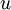is the relative velocity between the reference frames.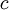is the speed of light (3x108 m s-1).

Evidence for time dilation was discovered by studying muon decay. Muons are subatomic particles with a short halflife of 1.53 microseconds. When produced by interactions of cosmic rays in the upper atmosphere, they have a speed around 0.994c. By putting muon detectors at the top (D1) and bottom (D2) of a mountain with a separation of 1900 m, scientists could measure accurately the proportion of muons reaching the second detector in comparison to the first. The proportion found was different to the proportion that was calculated without taking into account relativistic effects.

Using the equation for exponential decay, they could use this proportion to calculate the time taken for the muons to decay, relative to the muon. Then, using the time dilation equation they could then work out the dilated time. The dilated time showed a good correlation with the time it took the muons to reach the second sensor, thereby supporting the existence of time dilation.

The time taken for a muon to travel from D1 to D2 as measured by a stationary observer is: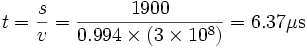The fraction of muons arriving at D2 in comparison to D1 was 0.732. (Given by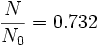)

Since (from the equation for exponential decay)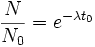thenThis gives the time for the proportion of decay to occur for an observer who is stationary, relative to the muon.

Putting this into the time dilation equation gives: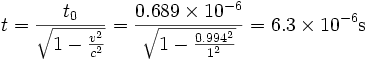This is in good agreement with the value calculated above, thereby providing evidence to support time dilation.

Since either reference frame is equally valid, from the muon's point of view it sees the earth approach it at nearly the speed of light. Hence time passes faster for the muon (slower for an observer on the ground). This appears to be a contradiction. However, the muon sees the height of the mountain contracted and so travels a shorter distance in its own frame. See length contraction below.

#### Time Dilation and Creation Science

For a more detailed treatment, see Starlight problem#Humphreys.27_model.

Creation scientists such as physicists Dr. Russell Humphreys and Dr. John Hartnett have used relativistic time dilation to explain how the earth can be only 6,000 years old even though cosmological data (background radiation, supernovae, etc.) set a much older age for the universe.

#### Derivation of Time Dilation

Time dilation is most easily derived using the Lorentz transformations, though geometrical solution is also straight forward. Using the transformation relating time between two frames of reference,and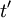. We can find the time difference between two events that occur at the same location in space. The events shall be called event one and event 2. This results in the equations: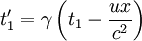whereis the Lorentz factoris the relative speed between reference framesis the speed of light

Subtracting the top equation from the bottom produces the time between the events as measured in each reference frame, so:This the equation for time dilation and is the same equation as earlier.

### Length contraction

When two inertial reference frames move past each other in a straight line with constant relative velocity, an observer in one reference frame would observe a metre rule in the other frame to be shorter along the direction parallel to the relative motion.

The length,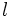, of an object as seen by a (relative) stationary observer is given by: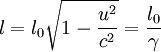Whereis the "proper length" or the length of the object in its own frame of reference.is the relative velocity between the reference frames.is the speed of lightm s-1is the Lorentz factor

#### Derivation

Length contraction may be derived using the Lorentz transformations as with time dilation. This time we use the equation for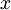. In this case, the time in the undashed frame must be the same. Following the same procedure as above we find that: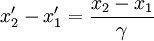This is the same as above withand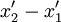being the lengths in the undashed and dashed frames respectively. Again, geometrical arguments may be used to achieve the same result.

### Apparent mass increase

For decades the theory of relativity taught that as a body moves with increasing velocity its mass also increases.

Under this view, the mass,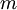, of an object as detected by a (relative) stationary observer is given by: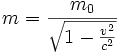Where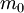is the "rest mass" or the mass of the object measured by an observer in the same reference frame as the object.is the relative velocity of the object.is the speed of light (3x108 ms-1).

Since speed is relative, it follows that two observers in different inertial reference frames may disagree on the mass and kinetic energy of a body. Since all inertial reference frames are treated on an equal footing, it follows that mass and energy are interchangeable.

In recent years most physicists have shifted away from Einstein's original reliance on relativistic mass and his suggestion that mass increases[Citation Needed]. Instead, most physicists today teach thatwhere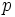is the momentum defined by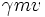is the standard Lorentz factoris the proper time

Force F defined this way is a vector and thus can handle the directional aspect of the relativistic effects better than the concept of relativistic mass can. The abandonment by physicists of the concept of relativistic mass, however, has the consequence of undermining the traditional claim under relativity thatalso popularly known asNow a concept of the 4-momentumof a particle is taught, such that the square of the magnitude ofsatisfies:in any inertial reference frame. The magnitude of the 4-momentum, in any inertial frame, equals the rest massof the particle (in units where).

## Relativity in everyday life

Due to the small speeds and gravitational fields in normal life, relativistic phenomena such as time dilation and length contraction are rarely observed. However some things in everyday life can be explained using relativity:

• GPS, the satellites experience time dilation due to the difference in speed and the strength of gravitational field between the satellite and the ground. This is corrected by daily synchronisation between the ground and the atomic clocks in the satellites.
• While most elemental metals such as silver, zinc and mercury have a silver/grey appearance, some metals like gold and copper do not. This difference can be explained using relativistic quantum mechanics.

The predictions of the theory of relativity throw up a number of apparent paradoxes and anomalies relating to the effects of time dilatation and length contraction. Whilst these paradoxes are consistent with the theory, they are contrary to everyday human experience and therefore can seem like impossibilities.

The twin paradox is usually stated as a thought experiment involving two twins, one of whom is sent on a long journey in a spacecraft travelling at close to the speed of light, whilst the other remains on Earth. Time dilatation means that the travelling twin, on his return to Earth, is younger that the twin who has remained at home. However, because neither twin is in a special position - each being in an inertial frame of reference - the reverse must also be true, and so the twin remaining on Earth must be younger. Hence each twin is younger than the other - a paradox.

The problem can be resolved in two ways. One is to examine the effects of General Relativity: to come back to Earth, the travelling twin must undergo acceleration in order to reverse his course, causing temporal effects which make him permanently the younger. Alternatively, it can be explained entirely using Special Relativity and noting that the twins are not in symmetrical situations: the one on earth has remained in a single inertial frame of reference, whilst the travelling twin has travelled in two. Note that the length of the trip cannot be increased as to make the acceleration negligible.

The Ehrenfest Paradox considers a rigid wheel or disc rotating a bout its axis at high speed (somewhat like a bicycle wheel spinning freely on its axle). The rim of the wheel travels at close to the speed of light and therefore undergoes length contraction, whereas the radius (the spokes, for the bicycle wheel) does not. Hence the circumference is no longer equal to 2r, which is paradoxical.

The apparent paradox was finally resolved in 1975 by the Norwegian scientist Øyvind Grøn.

This is not a paradox. It arises from failure to know or understand the "speed addition" formula. The formula tells how fast each observer measures the speed of the other observer when they are both traveling toward each other, as seen by some outside observer, at speedsand. Under classical mechanics, the result is just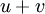. But the formula under special relativity isThis formula is a consequence of the Lorentz transform. It is a well known part of the basic undergraduate physics curriculum.

So, if two spacecraft are approaching each other, each traveling at what an outside observer would measure as half the speed of light, a person ignorant of how relativity works might think they each see the other coming toward them at the speed of light. But the formula shows that they actually see each other moving at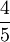of the speed of light.

Ifandare both less than the speed of light, one can show that the result of the addition formula will be also.

## Variable Speed of Light

The Theory of Relativity implies that physical constants like c, the speed of light in a vacuum, have remained constant. But at least one study suggests that physical constants, and possibly even the speed of light, have changed as the universe has aged.

"For the first time, scientists have experimentally demonstrated that sound pulses can travel at velocities faster than the speed of light, c. William Robertson's team from Middle Tennessee State University also showed that the group velocity of sound waves can become infinite, and even negative. ... Although such results may at first appear to violate special relativity (Einstein's law that no material object can exceed the speed of light), the actual significance of these experiments is a little different. These types of superluminal phenomena, Robertson et al. explain, violate neither causality nor special relativity, nor do they enable information to travel faster than c. In fact, theoretical work had predicted that the superluminal speed of the group velocity of sound waves should exist. 'The key to understanding this seeming paradox is that no wave energy exceeded the speed of light,' said Robertson."

"A team of researchers from the Ecole Polytechnique Fédérale de Lausanne (EPFL) has successfully demonstrated, for the first time, that it is possible to control the speed of light – both slowing it down and speeding it up – in an optical fiber, using off-the-shelf instrumentation in normal environmental conditions. Their results, to be published in the August 22 issue of Applied Physics Letters, could have implications that range from optical computing to the fiber-optic telecommunications industry." Both slowing down and speeding up of light within a substance other than a vacuum is made possible, because the light travels through the material, and that material affects the speed of light, i.e. a photon hits an electron, which then exits and emits a slightly lower energy photon out in the direction that the original photon was traveling, thus maintaining conservation of momentum. No matter how transparent an object may appear, it radically impacts the speed of the light traveling through it, as demonstrated by the refractive production of a rainbow by a crystal, which Newton himself discovered.

This apparent change in speed can be explained, however, by noting that the constant c refers to the speed of light in a vacuum, i.e., when it is unimpeded. The speed of light when traveling through physical media is, in fact, variable.

"A pair of German physicists claim to have broken the speed of light - an achievement that would undermine our entire understanding of space and time. ... Dr Nimtz told New Scientist magazine: 'For the time being, this is the only violation of special relativity that I know of.'"

## Pending research

Today some physicists are working on hypothesizing how general relativity might have related to the other three forces of nature during the first fraction of a second of the Big Bang. Two of the more commonly studied attempts are string theory and loop quantum gravity, but they have failed to produce any evidence that science mandates a science must have, and both typically take large amounts of work to even conform to what scientists believe. Critics increasingly point out that string theory and loop quantum gravity are largely untestable and unfalsifiable, and thus potentially unscientific under the principles of science advanced by Karl Popper.

Relativity continues to be tested and some physics professors remain skeptical of the theory, such as University of Maryland physics professor Carroll Alley, who served as the principle physicist on the Apollo lunar project.

## Political aspects of relativity

Some liberal politicians have extrapolated the theory of relativity to metaphorically justify their own political agendas. For example, Democratic President Barack Obama helped publish an article by liberal law professor Laurence Tribe to apply the relativistic concept of "curvature of space" to promote a broad legal right to abortion. As of June 2008, over 170 law review articles have cited this liberal application of the theory of relativity to legal arguments. Applications of the theory of relativity to change morality have also been common. Moreover, there is an unmistakable effort to censor or ostracize criticism of relativity.

Physicist Robert Dicke of Princeton University was a prominent critic of general relativity, and Dicke's alternative "has enjoyed a renaissance in connection with theories of higher dimensional space-time." Despite being one of the most accomplished physicists in the 20th century, Dicke was repeatedly passed over for a Nobel Prize, and in at least one case Dicke was insulted by the award being granted to others for contributions more properly credited to Dicke.

There has been little recognition by the Nobel Prize committee of either theory of relativity, and particularly scant recognition of the Theory of General Relativity. A dubious 1993 Nobel Prize in physics was awarded Hulse and Taylor for supposedly finding the first evidence of gravitational waves in the orbital decay of the binary pulsar PSR1913+16. A close reading of the paper reveals that that is based heavily on assumptions in trying to retrofit the data to the theory.

### Government Support for Relativistic research

The Federal Government has funded the building of two gravity wave detectors: The first to test the principle, and the second (upgrade) to actually perform measurements. As a result of this work, on February 11, 2016, the LIGO team reported successful detection of gravitational waves caused by the merging of two black holes.